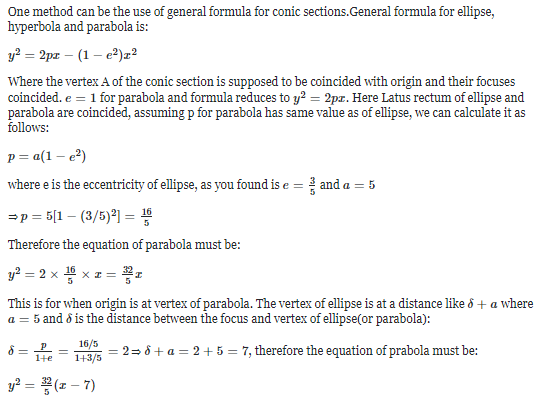×#### Thank you for registering.

One of our academic counsellors will contact you within 1 working day.

Click to Chat

1800-1023-196

+91-120-4616500

CART 0

• 0

MY CART (5)

Use Coupon: CART20 and get 20% off on all online Study Material

ITEM
DETAILS
MRP
DISCOUNT
FINAL PRICE
Total Price: Rs.

There are no items in this cart.
Continue Shopping
```
a particle of mass 0.002 kg and a charge 1 mewC is held at rest on a frictionless horizontal surfaces at a distance of 1 m from a fixed charge of 1mC .if the particle is relaxed,it will be repelled.the speed of the particle when it is at a distance of 10m from the fixed charge is- 60m/s 75m/s 90m/s 100m/s

```
6 months ago

```							mass of particle, m = 2g = 2 × 10^-3 kg charge on particle , q = 10^-6 C another charged particle Q = 1mC = 10^-3C is fixed at r = 1m from first particle. repulsion force acts between particles , F = kqQ/r² = 9 × 10^9 × 10^-3 × 10^-6/1² = 9N from Newton's 2nd law, Acceleration of first particle , a = F/m = 9/2 × 10^-3 = 9000/2 = 450 m/s² Let final velocity of particle is v m/s initial velocity of article is 0 m/s displacement covered by particle = final position - initial position = 10m - 1m = 9m using formula, v² = u² + 2as or, v² = 0 + 2 × 450 × 9 or, v² = 8100 = 90² => v = 90 m/s hence, velocity of particle it is at a distance of 10 m from the fixed charge is 90m/s
```
6 months agoSaurabh Koranglekar
8407 Points
``````
6 months ago
Think You Can Provide A Better Answer ?

## Other Related Questions on Organic Chemistry

View all Questions »### Course Features

• 731 Video Lectures
• Revision Notes
• Previous Year Papers
• Mind Map
• Study Planner
• NCERT Solutions
• Discussion Forum
• Test paper with Video Solution### Course Features

• 70 Video Lectures
• Revision Notes
• Test paper with Video Solution
• Mind Map
• Study Planner
• NCERT Solutions
• Discussion Forum
• Previous Year Exam Questions